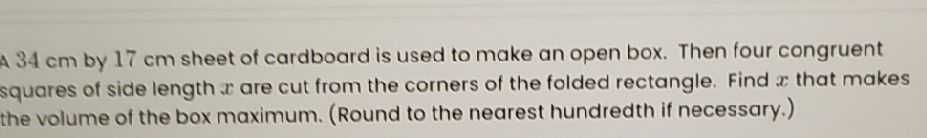### ¿Todavía tienes preguntas de matemáticas?

Pregunte a nuestros tutores expertos
Algebra
Pregunta$$34 cm$$ by $$17 cm$$ sheet of cardboard is used to make an open box. Then four congruent squares of side length $$x$$ are cut from the corners of the folded rectangle. Find $$x$$ that makes the volume of the box maximum. (Round to the nearest hundredth if necessary.)